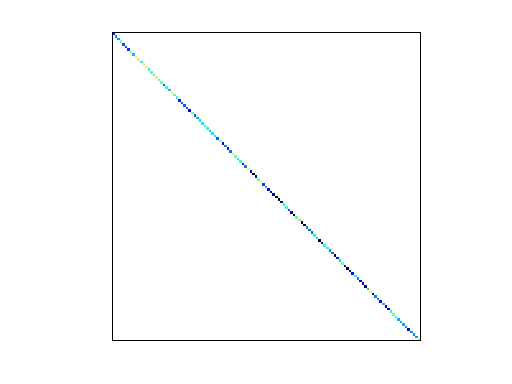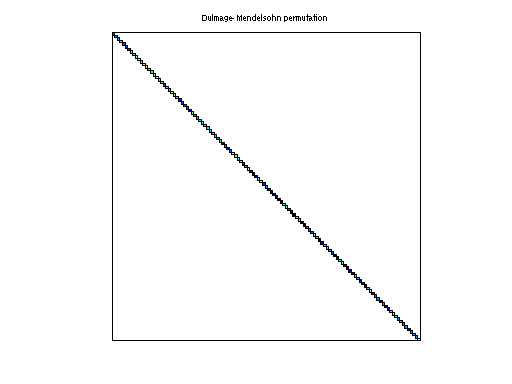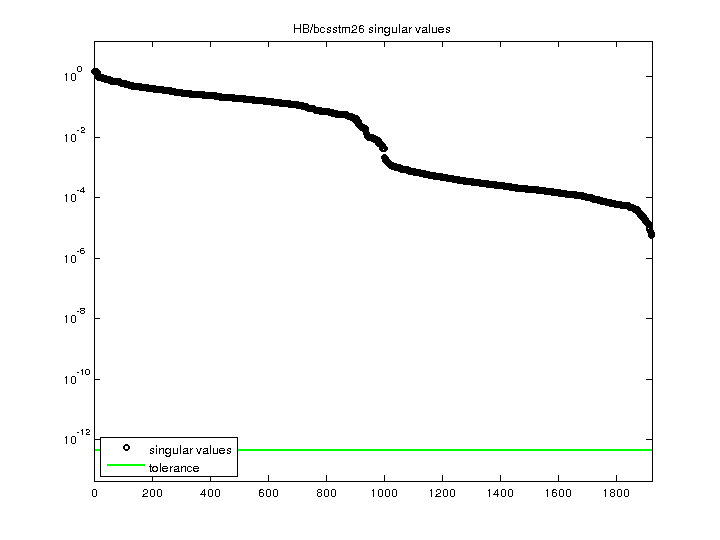Matrix: HB/bcsstm26

Description: SYMMETRIC MASS MATRIX - REACTOR CONTAINMENT FLOOR -- SECTION(undirected graph drawing)• Matrix group: HB
• download as a MATLAB mat-file, file size: 14 KB. Use UFget(76) or UFget('HB/bcsstm26') in MATLAB.

 Matrix properties number of rows 1,922 number of columns 1,922 nonzeros 1,922 structural full rank? yes structural rank 1,922 # of blocks from dmperm 1,922 # strongly connected comp. 1,922 explicit zero entries 0 nonzero pattern symmetry symmetric numeric value symmetry symmetric type real structure symmetric Cholesky candidate? yes positive definite? yes

 author A. Mera, R. Cigel, J. Lewis editor I. Duff, R. Grimes, J. Lewis date 1984 kind structural problem 2D/3D problem? yes

 Ordering statistics: result nnz(chol(P*(A+A'+s*I)*P')) with AMD 1,922 Cholesky flop count 1.9e+03 nnz(L+U), no partial pivoting, with AMD 1,922 nnz(V) for QR, upper bound nnz(L) for LU, with COLAMD 1,922 nnz(R) for QR, upper bound nnz(U) for LU, with COLAMD 1,922

 SVD-based statistics: norm(A) 1.5124 min(svd(A)) 5.7821e-06 cond(A) 261567 rank(A) 1,922 sprank(A)-rank(A) 0 null space dimension 0 full numerical rank? yes

 singular values (MAT file): click here SVD method used: s = svd (full (A)) ; status: ok